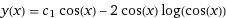# Differential Equations: Solve the following

• komarxian
If you want to find the integrating factor ##p(x)##, you should use the following formula:$$p(x) = \frac{e^{\ln(\cos x)}}{e^{\ln(\cos x + k)}}$$ where ##k## is a constant. In summary, you made a sign error when finding the integrating factor ##p(x)##, and you should use the following formula to find it.f

## Homework Statement

Solve the following differential equations/initial value problems:

(cosx) y' + (sinx) y = sin2x

## Homework Equations

I've been attempting to use the trig ID sin2x = 2sinxcosx.
I am also trying to solve this problem by using p(x)/P(x) and Q(x)

## The Attempt at a Solution

[/B]
cosx y' +sinx y = 2sinxcosx

y' + tanx y = 2 sinx

P(x) = tanx, Q(x) = 2sinx

--> p(x) = e^lncosx + c = e^ lncosx e^c = C cos x (if e^c = C)

Dx = p(x)Q(x) = Ccosx (2sinx) = c 2 sinxcosx = Csin2x

p(x) y(x) = int( Dx ) dx = int( Csin2x) dx = C -cos2x/2 + C

y(x) = C -cos2x/2(Ccosx) + C/Ccosx = -Ccos2x/2(Ccosx) + 1/cosx = -Ccos2x/2(Ccosx) + secx

I'm kind of confused, because my constant C disappears at some point, and the answer is supposed to beany recommendations as to where I went wrong?

## Homework Statement

Solve the following differential equations/initial value problems:

(cosx) y' + (sinx) y = sin2x

## Homework Equations

I've been attempting to use the trig ID sin2x = 2sinxcosx.
I am also trying to solve this problem by using p(x)/P(x) and Q(x)

## The Attempt at a Solution

[/B]
cosx y' +sinx y = 2sinxcosx

y' + tanx y = 2 sinx

P(x) = tanx, Q(x) = 2sinx

--> p(x) = e^lncosx + c = e^ lncosx e^c = C cos x (if e^c = C)

Dx = p(x)Q(x) = Ccosx (2sinx) = c 2 sinxcosx = Csin2x

p(x) y(x) = int( Dx ) dx = int( Csin2x) dx = C -cos2x/2 + C

y(x) = C -cos2x/2(Ccosx) + C/Ccosx = -Ccos2x/2(Ccosx) + 1/cosx = -Ccos2x/2(Ccosx) + secx

I'm kind of confused, because my constant C disappears at some point, and the answer is supposed to be
View attachment 228687

any recommendations as to where I went wrong?

If ##y'(x) + p(x) y(x) = q(x),## the solution is of the form
$$y(x) = e^{-P(x)} \int_0^x q(t) e^{P(t)} \, dt + c e^{-P(x)},$$
where ##P(w) = \int_0^w p(u) \, du## for any ##w##.

Note that if we include another constant of integration alongside ##P## (so we replace ##P(w)## by ##P(w)+k## for a constant ##k##) the constant cancels in the first term, and replaces ##c## by ##c e^{-k} = \text{another constant}## in the second term.

•komarxian
y' + tanx y = 2 sinx

P(x) = tanx, Q(x) = 2sinx

--> p(x) = e^lncosx + c = e^ lncosx e^c = C cos x (if e^c = C)
You made a sign error when finding the integrating factor ##p(x)##. If you differentiate ##\log \cos x##, you get ##-\tan x##.

Also, if you differentiate ##y \cos x##, you get ##(\cos x) y' + (-\sin x) y##, which isn't the lefthand side of the DE you started with.